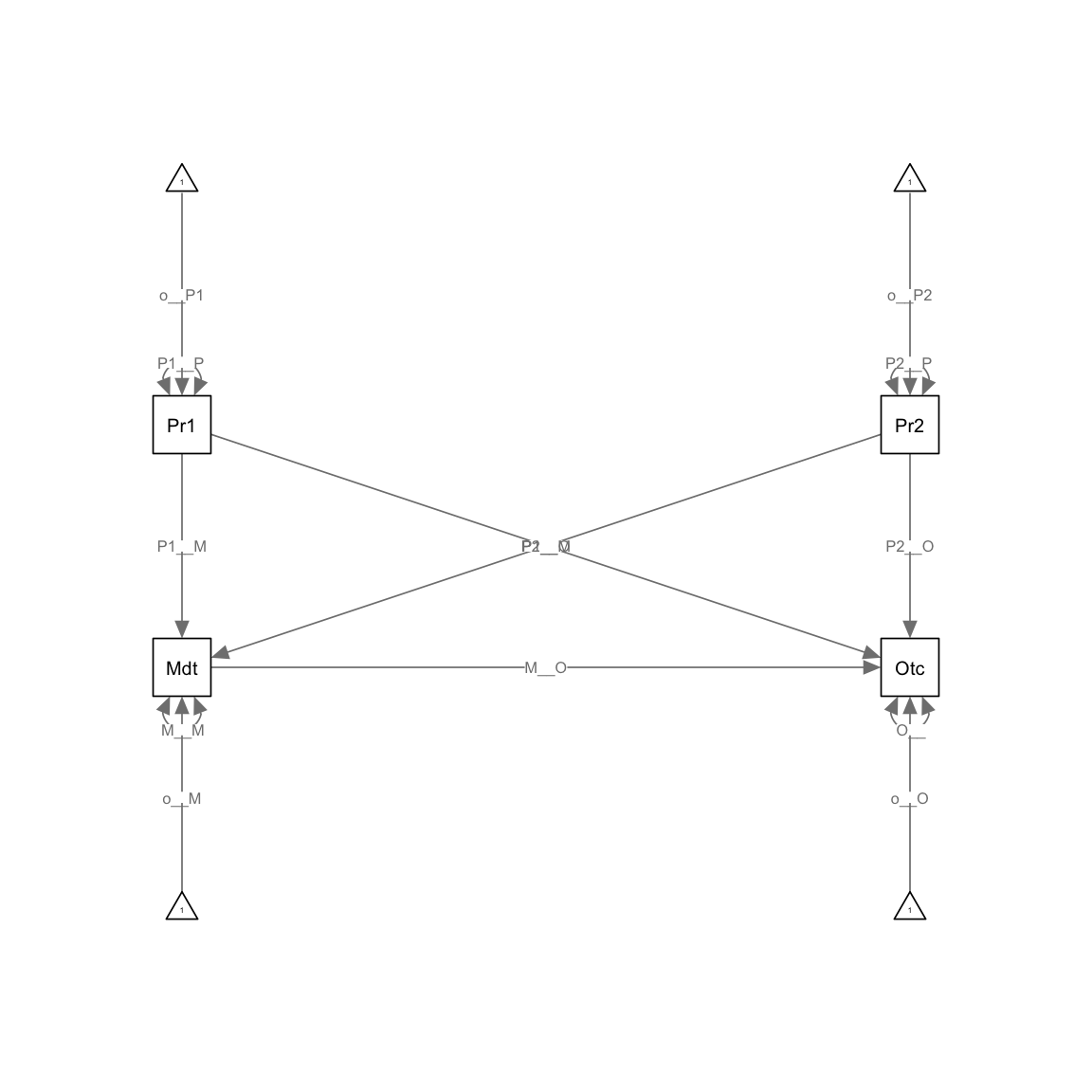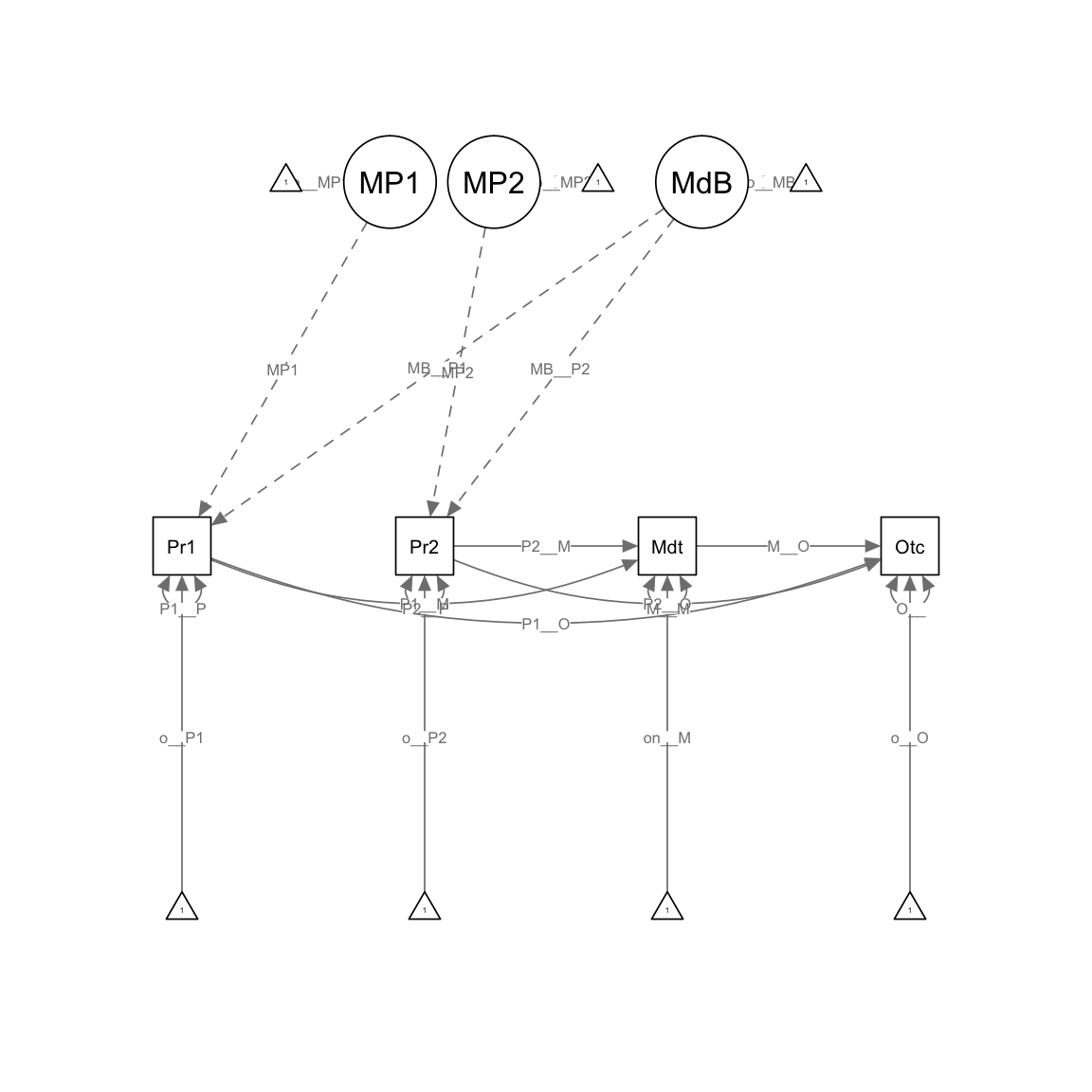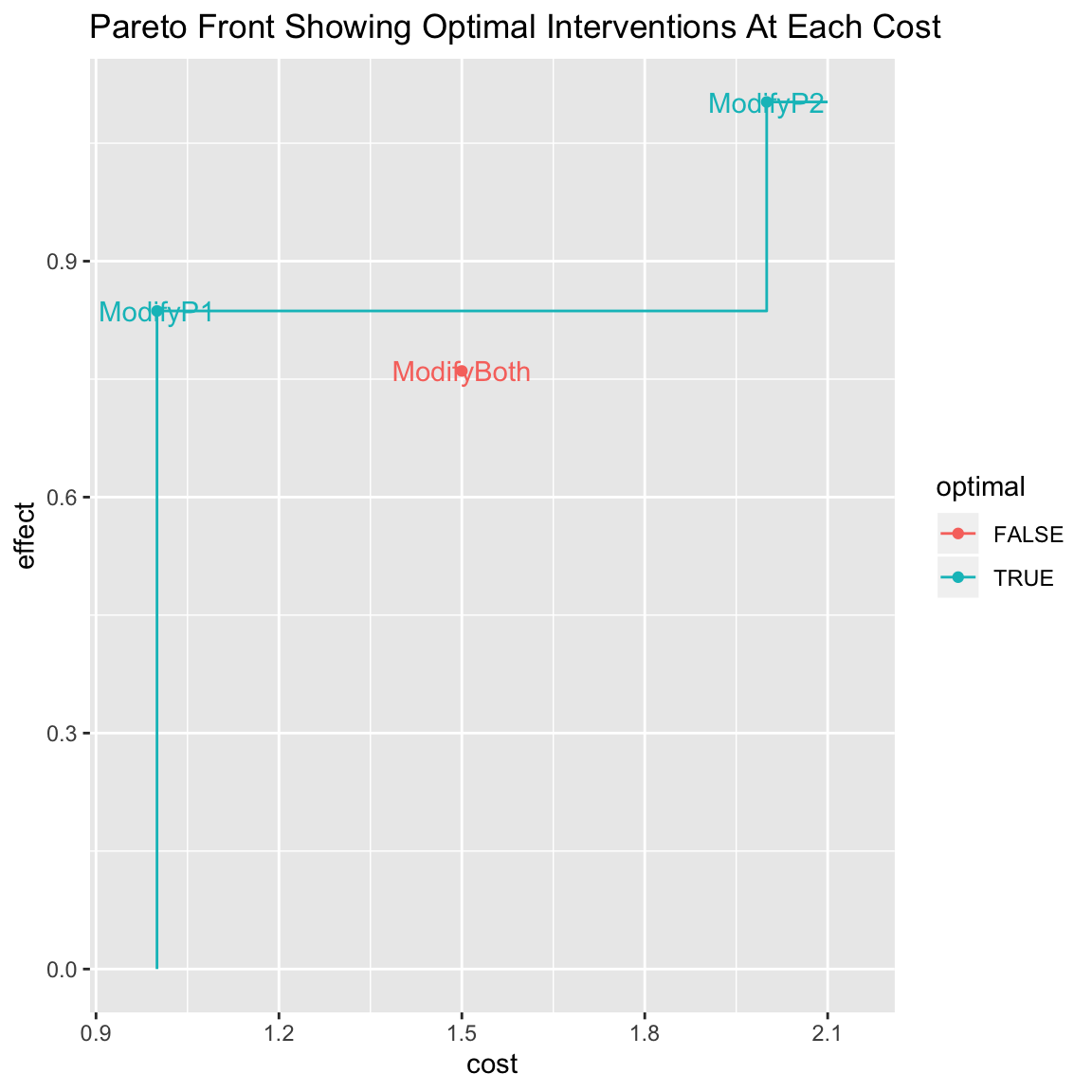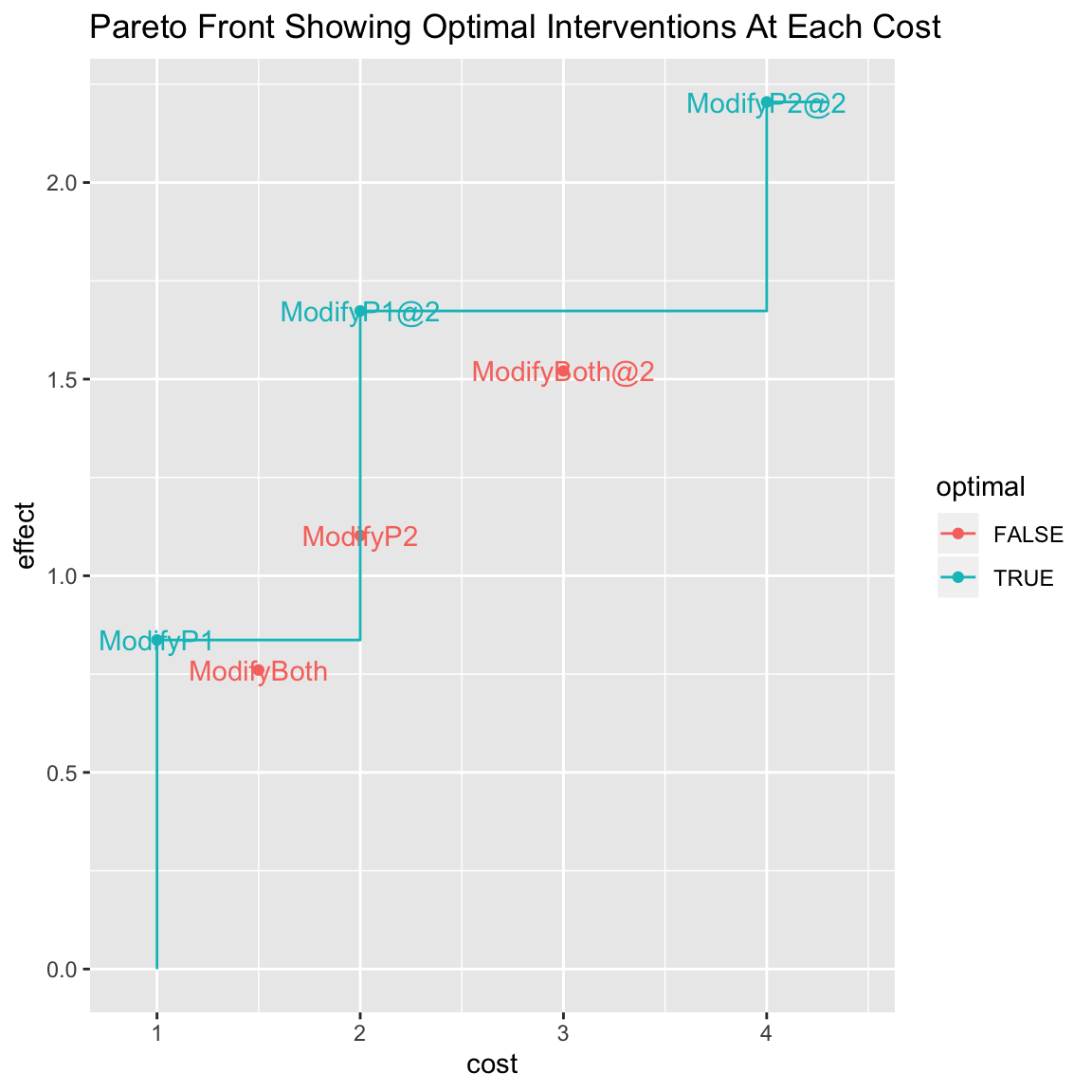1. Introduction
2. Mediation model MICs
3. Potential Intervention Evaluation
4. Multiple Objective Optimization

## Introduction

In this vignette, we provide an introduction to using MICs to select among a set of interventions. We assume the mediation model described in Brick & Bailey (submitted), in which Z partially mediates the effect of X on Y, and in which there are several approaches for

``````library(MICr)
library(umx)
library(knitr)
drawPlots <- library(semPlot, logical.return = TRUE)  # Plot only if there's a plotting function.
knitr::opts_chunk\$set(fig.align="center")``````

## Mediation Model MICs

We can construct a multiple mediator data set with a few quick lines of R code:

``````set.seed(1234)
# Predictors
Predictor1 <- rnorm(100)
Predictor2 <- rnorm(100)
# Mediator
Mediator  <- .3*Predictor1 + .7*Predictor2 + rnorm(100)
#Outcome
Outcome  <-0*Predictor1 + 0.4*Predictor2 + .7*Mediator + rnorm(100)
# Combined Data
theData <- data.frame(Predictor1, Predictor2, Mediator, Outcome)``````

We’ll start out by just building the mediation model. We’ll use `umx` here for simplicity and `semPaths` for plotting.

``````model <- umxRAM("MediationModel", data=theData,
# Predictor Effects
umxPath(fromEach=c("Predictor1", "Predictor2"), to=c("Mediator", "Outcome")),

# Mediator Effect
umxPath(from="Mediator", to="Outcome"),
# Variances, means, and intercepts
umxPath(v.m. = c("Predictor1", "Predictor2", "Mediator", "Outcome"))
)``````
name Estimate SE
Predictor1_to_Mediator 0.381 0.095
Predictor1_to_Outcome 0.143 0.110
Predictor2_to_Mediator 0.788 0.092
Predictor2_to_Outcome 0.533 0.131
Mediator_to_Outcome 0.723 0.108
Predictor1_with_Predictor1 0.999 0.141
Predictor2_with_Predictor2 1.055 0.149
Mediator_with_Mediator 0.898 0.127
Outcome_with_Outcome 1.046 0.148
one_to_Predictor1 -0.157 0.100
one_to_Predictor2 0.041 0.103
one_to_Mediator 0.164 0.096
one_to_Outcome 0.006 0.105

 “χ²(1) = 0.06, p = 0.800; CFI = 1.006; TLI = 1.035; RMSEA = 0”

``if(drawPlots) semPaths(model)``We can generate the causal implications of this model by computing the MIC. This table shows the model-implied causal effects of each variable on each other variable. These are loading weights for cases where there is only a direct effect, and total effects when there are both direct and indirect effects.

``MIC(model)``
``````##            Predictor1 Predictor2  Mediator Outcome
## Predictor1  1.0000000  0.0000000 0.0000000       0
## Predictor2  0.0000000  1.0000000 0.0000000       0
## Mediator    0.3805142  0.7883193 1.0000000       0
## Outcome     0.4183552  1.1024172 0.7226811       1``````

We can format them more precisely (and with standard errors) using the `MICTable()` function:

``MICTable(model, se=TRUE)``
from to MediationModel MediationModel_SE
Predictor2 Outcome 1.1024172 0.1198877
Predictor2 Mediator 0.7883193 0.0923177
Mediator Outcome 0.7226811 0.1078953
Predictor1 Outcome 0.4183552 0.1232033
Predictor1 Mediator 0.3805142 0.0948715

## Evaluating potential interventions

In this case, we will examine the effects of three different potential interventions on the outcome: one intervention (`ModifyP1`) that improves `Predictor1` by two points, another (`ModifyP2`) that increases `Predictor2` by one point, and a joint intervention `ModifyBoth` that increases both by a half point.

``````ixnModel <- umxRAM("MediationModel", data=theData,
# Predictor Effects
umxPath(fromEach=c("Predictor1", "Predictor2"), to=c("Mediator", "Outcome")),

# (Latent) Intervention Effects
umxPath(from="ModifyP1", to="Predictor1", fixedAt=2.0),
umxPath(from="ModifyP2", to="Predictor2", fixedAt=1.0),
umxPath(from="ModifyBoth", to=c("Predictor1", "Predictor2"), fixedAt=c(.5, .5)),

# Mediator Effect
umxPath(from="Mediator", to="Outcome"),
# Variances, means, and intercepts
umxPath(v.m. = c("Predictor1", "Predictor2", "Mediator", "Outcome"))
)``````
name Estimate SE
Predictor1_to_Mediator 0.381 0.095
Predictor1_to_Outcome 0.143 0.110
Predictor2_to_Mediator 0.788 0.092
Predictor2_to_Outcome 0.533 0.131
Mediator_to_Outcome 0.723 0.108
ModifyP1_to_Predictor1 2.000 0.000
ModifyP2_to_Predictor2 1.000 0.000
ModifyBoth_to_Predictor1 0.500 0.000
ModifyBoth_to_Predictor2 0.500 0.000
Predictor1_with_Predictor1 0.999 0.141
Predictor2_with_Predictor2 1.055 0.149
Mediator_with_Mediator 0.898 0.127
Outcome_with_Outcome 1.046 0.148
one_to_Predictor1 -0.157 0.100
one_to_Predictor2 0.041 0.103
one_to_Mediator 0.164 0.096
one_to_Outcome 0.006 0.105

 “χ²(1) = 0.06, p = 0.800; CFI = 1.006; TLI = 1.035; RMSEA = 0”

``if(drawPlots) semPaths(ixnModel)``The intervention model’s MIC looks like this:

``MIC(ixnModel)``
``````##            Predictor1 Predictor2  Mediator Outcome  ModifyP1  ModifyP2
## Predictor1  1.0000000  0.0000000 0.0000000       0 2.0000000 0.0000000
## Predictor2  0.0000000  1.0000000 0.0000000       0 0.0000000 1.0000000
## Mediator    0.3805142  0.7883193 1.0000000       0 0.7610285 0.7883193
## Outcome     0.4183552  1.1024172 0.7226811       1 0.8367105 1.1024172
## ModifyP1    0.0000000  0.0000000 0.0000000       0 1.0000000 0.0000000
## ModifyP2    0.0000000  0.0000000 0.0000000       0 0.0000000 1.0000000
## ModifyBoth  0.0000000  0.0000000 0.0000000       0 0.0000000 0.0000000
##            ModifyBoth
## Predictor1  0.5000000
## Predictor2  0.5000000
## Mediator    0.5844167
## Outcome     0.7603862
## ModifyP1    0.0000000
## ModifyP2    0.0000000
## ModifyBoth  1.0000000``````

The MIC is somewhat intricate, so we’ll again use the MICTable format:

``MICTable(ixnModel, caption="Intervention Results")``
from to MediationModel MediationModel_SE
ModifyP1 Predictor1 2.0000000 0.0000000
Predictor2 Outcome 1.1024172 0.1198877
ModifyP2 Outcome 1.1024172 0.1198877
ModifyP2 Predictor2 1.0000000 0.0000000
ModifyP1 Outcome 0.8367105 0.2464065
Predictor2 Mediator 0.7883193 0.0923177
ModifyP2 Mediator 0.7883193 0.0923177
ModifyP1 Mediator 0.7610285 0.1897431
ModifyBoth Outcome 0.7603862 0.0870376
Mediator Outcome 0.7226811 0.1078953
ModifyBoth Mediator 0.5844167 0.0670219
ModifyBoth Predictor1 0.5000000 0.0000000
ModifyBoth Predictor2 0.5000000 0.0000000
Predictor1 Outcome 0.4183552 0.1232033
Predictor1 Mediator 0.3805142 0.0948715

Even here, there’s complexity. It can be helpful to look directly at the effects of the interventions on the outcomes:

``MICTable(ixnModel, caption="Implied Intervention Effects On Output", from=c("ModifyP1", "ModifyP2", "ModifyBoth"), to="Outcome")``
from to MediationModel MediationModel_SE
ModifyP2 Outcome 1.1024172 0.1198877
ModifyP1 Outcome 0.8367105 0.2464065
ModifyBoth Outcome 0.7603862 0.0870376

ModifyP2 has the strongest predicted influence on the outcome, partially because the direct effects of P2 are so strong. In case the mediator is also of interest, we can check the influence on that as well.

``MICTable(ixnModel, caption="Implied Intervention Effects On Output", from=c("ModifyP1", "ModifyP2", "ModifyBoth"), to="Mediator")``
from to MediationModel MediationModel_SE
ModifyP2 Mediator 0.7883193 0.0923177
ModifyP1 Mediator 0.7610285 0.1897431
ModifyBoth Mediator 0.5844167 0.0670219

If we’re interested in modifying both the mediator and the outcome, ModifyP1 may be a better choice. If we are interested in modifying only the outcome, ModifyP2 is better.

## Multiobjective Optimization

In cases with many possible outcomes, it may be helpful to generate a pareto plot that balances the cost against the influence of the outcome. A Pareto plot provides a tradeoff surface showing the maximum influence of outcome at a given level of cost. We can do this by assigning costs to each of the interactions in the MIC Table for the outcome.

The cost function here is fairly simple:

``````costFrame <- data.frame(name=c("ModifyP1", "ModifyP2", "ModifyBoth"), cost=c(1, 2, 1.5))
costFrame``````
``    name cost``

1 ModifyP1 1.0 2 ModifyP2 2.0 3 ModifyBoth 1.5

And then we can compute the pareto front and plot it directly from the MIC package.

``paretoFront(mic=ixnModel, outcome="Outcome", costs=costFrame)````````##   .DisplayName       name cost    effect optimal
## 2     ModifyP1   ModifyP1  1.0 0.8367105    TRUE
## 1   ModifyBoth ModifyBoth  1.5 0.7603862   FALSE
## 3     ModifyP2   ModifyP2  2.0 1.1024172    TRUE``````

To find the optimal effect at a given level of cost, trace upward to the line at the selected cost level, and then follow the line left to the first observed value. That represents the intervention with the largest effect on the outcome that can be achieved for that cost. If several have a common effect size, the optimal is the one with the lowest cost.

For better comparisons, we can add a `scale` column, for cases where the intervention may have a quantitative rather than discrete effect, e.g. where more intervention (e.g. more clinical sessions) is expected to have a linear causal effect on the outcome.

``````costFrame <- data.frame(name=c("ModifyP1", "ModifyP2", "ModifyBoth", "ModifyP1", "ModifyP2", "ModifyBoth"), cost=c(1, 2, 1.5, 2, 4, 3), scale=c(1,1,1,2,2,2))
costFrame``````
``````##         name cost scale
## 1   ModifyP1  1.0     1
## 2   ModifyP2  2.0     1
## 3 ModifyBoth  1.5     1
## 4   ModifyP1  2.0     2
## 5   ModifyP2  4.0     2
## 6 ModifyBoth  3.0     2``````

Here, the pareto front may be more informative:

``paretoFront(mic=ixnModel, outcome="Outcome", costs=costFrame)````````##   .DisplayName       name cost    effect optimal
## 3     ModifyP1   ModifyP1  1.0 0.8367105    TRUE
## 1   ModifyBoth ModifyBoth  1.5 0.7603862   FALSE
## 4   ModifyP1@2   ModifyP1  2.0 1.6734209    TRUE
## 5     ModifyP2   ModifyP2  2.0 1.1024172   FALSE
## 2 ModifyBoth@2 ModifyBoth  3.0 1.5207724   FALSE
## 6   ModifyP2@2   ModifyP2  4.0 2.2048343    TRUE``````

Here, it is clear that the `ModifyBoth` intervention is sub-optimal. Indeed, `ModifyP1` is so efficient that it is better to apply it with double effect than to apply `ModifyP2` at scale 1. The cost for these two interventions is the same, but the effect is stronger for `ModifyP1`.

Note that the `scale` here is a multiplier for the effect, not for the intervention. That is, it may take